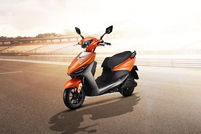# YObykes Yo Edge EMI CalculatorRs. 49,000*
*Ex-showroom Price in delhi

YObykes Yo Edge EMI starts at Rs 1,578 per month for a tenure of 36 months @9.7 for a loan amount of Rs 44,100. The EMI Calculator tool on BikeDekho gives a detailed break-up of the total payable amount and helps you in finding the best bike finance for your Edge. (Calculated on On Road Price in Delhi)

## YObykes Yo Edge Down Payment and EMI

Edge VariantsLoan @ 9.7%. Down paymentEMI (36 months)
STD44,100Rs. 4,900Rs. 1,578
Read More

## Calculate your Loan EMI for Edge

Down PaymentRs.
0Rs.0
Bank Interest Rate %
8%26%
Loan Period ( Months )
• On-Road PriceRs.0
• Total Loan AmountRs.0
• Payable AmountRs.0
• You’ll pay extraRs.0
EMIper month
Rs0
Calculated on On Road Price in delhi
At BikeDekho, We can help you get the best deal on your loans. Please call us on 1800 200 3000 for help.

## Find EMI of Edge Alternatives

*Ex-showroom Price in delhi

## Edge EMI FAQs

• FAQ

### What will be the lowest down payment for Yo Edge?

The lenders generally finance 90% of the value of the . Some customers might be eligible for 100% funding too. The down payment is the difference between the on-road price of the and the amount funded by a lender. This means the minimum possible down payment that you have to pay for Yo Edge includes the RTO and insurance charges.

### What will be the monthly EMI for Yo Edge?

EMIs or Equated Monthly Installments refer to the monthly payments you make to the lender to repay your loan. These payments include the principal amount as well as the interest i.e. EMI = Principal Amount + Interest on Principal amount. Mathematically, EMI for Yo Edge can be calculated using the following formula:

{P x R x (1+R)^N / [(1+R)^N-1]}

where, P = Principal amount of the loan, R = Rate of interest and N = Number of monthly installments.

For Example:- If the principal amount for a bike loan is Rs. ₹44,000/- on an annual rate of interest of 10% for a tenure of 3 years then

EMI = 44000 * 0.008333*(1+ 0.008333)^36 / ((1+ 0.008333)^36)-1 = Rs /-

The rate of interest (R) on your loan is calculated monthly i.e. (R= Annual rate of interest/12/100). For instance, if R = 10% per annum, then R= 10%/12 = 0.008333.

### What will be the interest rate for Yo Edge?

The interest rate for Yo Edge primarily depends on the principal amount and tenure of the loan amount. Interest rate of lenders generally varies from 9.7% per annum to 15% per annum. Customers can also negotiate with the financier for a better rate of interest against their loan amount.

### What will be the EMI for 3 years tenure for Yo Edge?

EMI for Yo Edge for 3 years tenure can be calculated as per the following formula:-

{P x R x (1+R)^N / [(1+R)^N-1]}

Where, P = Principal amount of the loan, R = Rate of interest, N = Number of monthly installments which will be 36 in case of 3 years tenure.

## Top 10 Scooters in India

*Ex-showroom Price in delhi

## Trending YObykes Scooters

*Ex-showroom price in Delhi

## Edge Running Cost

KM driven per day20 km/day
Monthly fuel costRs.0* / month
Disclaimer :

Disclaimer : As per the information entered by you the calculation is performed by EMI Calculator and the amount of installments does not includes any other fees charged by the financial institution / banks like processing fee, file charges, etc. The amount is in Indian Rupee rounded off to the nearest Rupee. Depending upon type and use of vehicle, regional lender requirements and the strength of your credit, actual down payment and resulting monthly payments may vary. Exact monthly installments can be found out from the financial institution.

Read More
×
We need your city to customize your experience